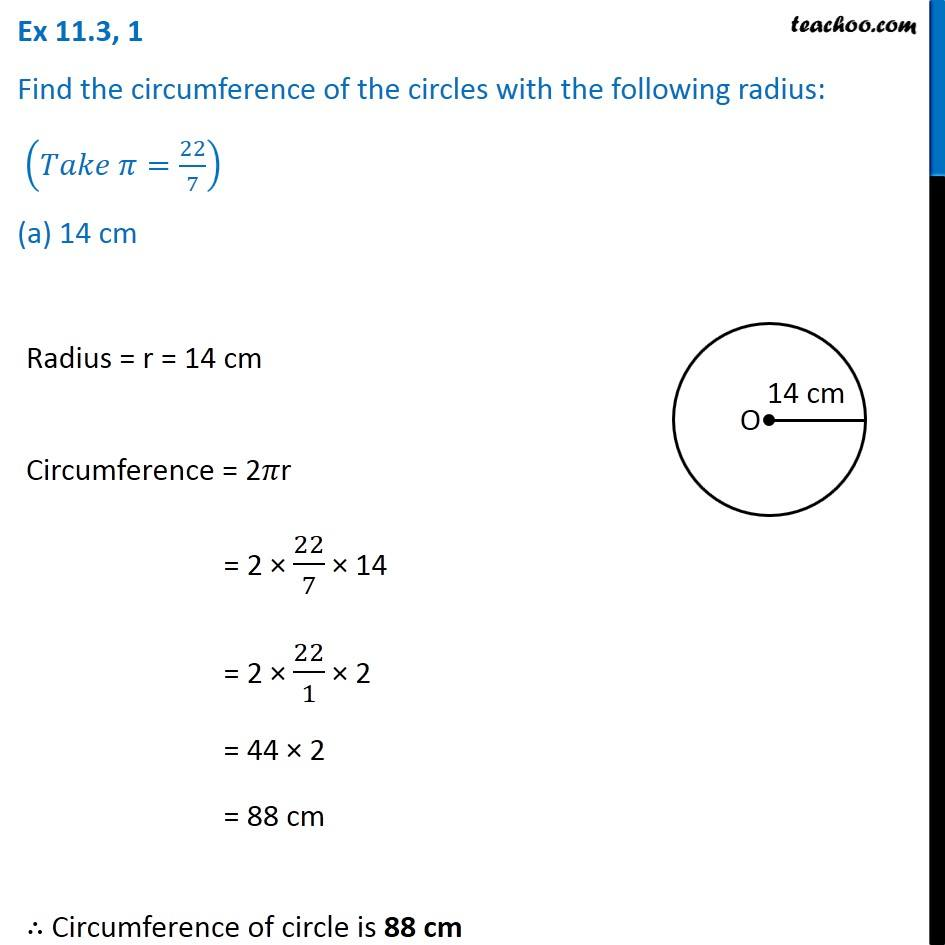1. Chapter 11 Class 7 Perimeter and Area
2. Concept wise
3. Circumference of circle

Transcript

Ex 11.3, 1 Find the circumference of the circles with the following radius: (𝑇𝑎𝑘𝑒 𝜋=22/7) (a) 14 cm Radius = r = 14 cm Circumference = 2𝜋r = 2 × 22/7 × 14 = 2 × 22/1 × 2 = 44 × 2 = 88 cm ∴ Circumference of circle is 88 cm